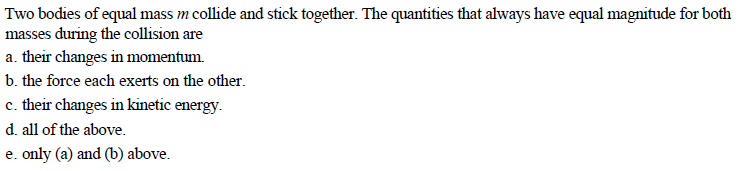# Two bodies of equal mass m collide and stick together. The quantities that always have equal magnitude for bothmasses during the collision area. their changes in momentumb. the force each exerts on the other.c. their changes in kinetic energy.d. all of the above.e. only (a) and (b) above.

Question
422 viewshelp_outlineImage TranscriptioncloseTwo bodies of equal mass m collide and stick together. The quantities that always have equal magnitude for both masses during the collision are a. their changes in momentum b. the force each exerts on the other. c. their changes in kinetic energy. d. all of the above. e. only (a) and (b) above. fullscreen
check_circle

Step 1

The change in momentum is having equal magnitude and oppositely directed.

Step 2

The forces are obeying Newton’s third law. So, the forces are equal and opposite.

The collision is inelastic collision. Thus, there will be loss in kinetic energy.

Step 3

Thus, only (a) and (b) a...

### Want to see the full answer?

See Solution

#### Want to see this answer and more?

Solutions are written by subject experts who are available 24/7. Questions are typically answered within 1 hour.*

See Solution
*Response times may vary by subject and question.
Tagged in
SciencePhysics

### Collisions